Go to: Related nodes. Attributes.

This node will insert knots at a particular parameter value(s). An edit point will appear where you insert the knot. Also, the number of spans and CVs in the curve will increase in the area where the knot is inserted. You can insert up to "degree" knots at a curve parameter that isn't already an edit point. eg. for a degree three curve, you can insert up to 3 knots. The more knots inserted at a parameter (default is 1), the more "pull" the new CVs have. eg. if you insert 3 knots into a degree 3 curve, one of the new CVs will have the curve passing directly through it.

Use this operation if you need more CVs in a local area of the curve. Use this operation (or "hardenPoint") if you want to create a corner in a curve.

This node is also useful if you have aligned an end of the curve at a specific tangent and you want the ability to change the curve shape near the end, while still maintaining the tangent. In this case, you can insert a knot near (but not at) the end, then leave last two CVs (which define the tangent) where they are, and modify any other CV to change the curve shape.

Node nameParentsMFn typeCompatible function sets
insertKnotCurveabstractBaseCreatekInsertKnotCrvkBase
kNamedObject
kDependencyNode
kCreate
kInsertKnotCrv

## Attributes (6)

Long name (short name)TypeDefaultFlags
`inputCurve` (`ic`) nurbsCurveNULL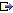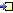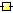The input curve
`parameter` (`p`) double0.0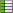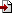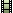Parameter value(s) where knots are added
`numberOfKnots` (`nk`) integer1How many knots to insert. At any point on the curve, there can be a maximum of "degree" knots.
`addKnots` (`add`) booltrueWhether to add knots or complement. Complement means knots will be added to reach the specified number of knots.
`insertBetween` (`ib`) boolfalseIf set to true, and there is more than one parameter value specified, the knots will get inserted at equally spaced intervals between the given parameter values, rather than at the parameter values themselves.
`outputCurve` (`oc`) nurbsCurveNULLThe output curve